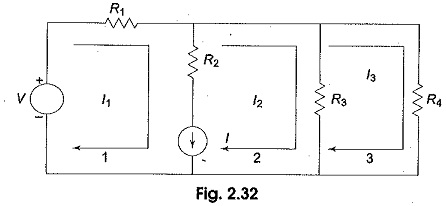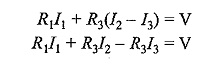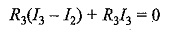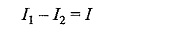## Super Mesh Circuit Analysis:

Suppose any of the branches in the network has a current source, then it is slightly difficult to apply mesh analysis straight forward because first we should assume an unknown voltage across the current source, writing mesh equations as before, and then relate the source current to the assigned mesh currents. This is generally a difficult approach. One way to overcome this difficulty is by applying the Super Mesh Circuit Analysis. Here we have to choose the kind of supermesh.

A Super Mesh Circuit Analysis is constituted by two adjacent loops that have a common current source. As an example, consider the network shown in Fig. 2.32.

Here, the current source I is in the common boundary for the two meshes 1 and 2. This current source creates a supermesh, which is nothing but a combination of meshes 1 and 2.Considering mesh 3, we haveFinally, the current I from current source is equal to the difference between two mesh currents, i.e.We have, thus, formed three mesh equations which we can solve for the three unknown currents in the network.

Scroll to Top